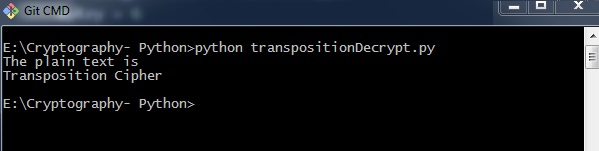# Decryption of Transposition Cipher

In this chapter, you will learn the procedure for decrypting the transposition cipher.

## Code

Observe the following code for a better understanding of decrypting a transposition cipher. The cipher text for message Transposition Cipher with key as 6 is fetched as Toners raiCntisippoh.

import math, pyperclip
def main():
myMessage= 'Toners raiCntisippoh'
myKey = 6
plaintext = decryptMessage(myKey, myMessage)

print("The plain text is")
print('Transposition Cipher')

def decryptMessage(key, message):
numOfColumns = math.ceil(len(message) / key)
numOfRows = key
numOfShadedBoxes = (numOfColumns * numOfRows) - len(message)
plaintext = float('') * numOfColumns
col = 0
row = 0

for symbol in message:
plaintext[col] += symbol
col += 1
if (col == numOfColumns) or (col == numOfColumns - 1 and row >= numOfRows - numOfShadedBoxes):
col = 0 row += 1 return ''.join(plaintext)
if __name__ == '__main__':
main()


### Explanation

The cipher text and the mentioned key are the two values taken as input parameters for decoding or decrypting the cipher text in reverse technique by placing characters in a column format and reading them in a horizontal manner.

You can place letters in a column format and later combined or concatenate them together using the following piece of code −

for symbol in message:
plaintext[col] += symbol
col += 1

if (col == numOfColumns) or (col == numOfColumns - 1 and row >= numOfRows - numOfShadedBoxes):
col = 0
row += 1
return ''.join(plaintext)


## Output

The program code for decrypting transposition cipher gives the following output −